Caided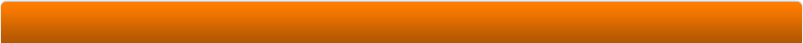# Program DetailsOn the first tab the sea level pressure and temperatures are entered allowing the density to be calculated.

From this having entered an altitude the pressure, temperature and density can be calculated at this altitudeThe drop down combo box can be pre-populated with values by using the pick lists dialogue box.

Shown here, a lower bound of 0 and an upper of 20000 has been set with a step value of 500. Having clicked generate, the list is created and this can then be applied to the Altitude drop down entries.The second tab, using the previous tab information calculates the stagnation conditions for a given flight speed

Either Velocity or a Mach number can be entered.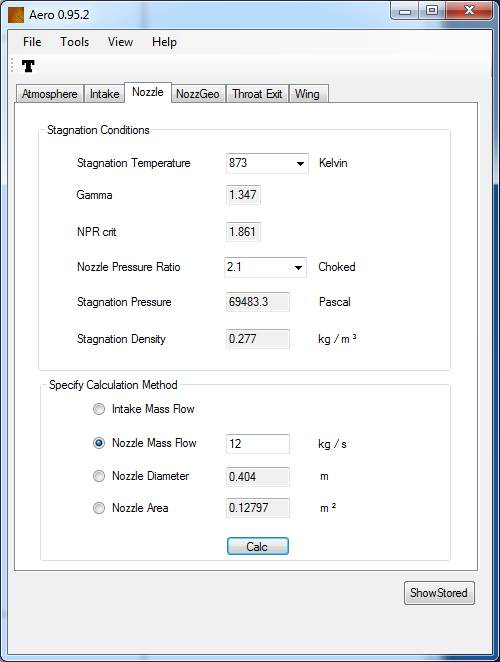The third tab using the previous conditions, for a given nozzle stagnation temperature and nozzle pressure ratio can calculate the nozzle exit area having specified the nozzle mass flow.
Ideal gas, isentropic equations are used suitable for compressible flow calculations.
The ratio of specific heats of the gas is automatically calculated for a given resevoir temperature. This is iteratively re-calculated for the static conditions at the throat.

The inverse calculation can be done by specifying the nozzle exit area and the program calculates the mass flow or a nozzle area.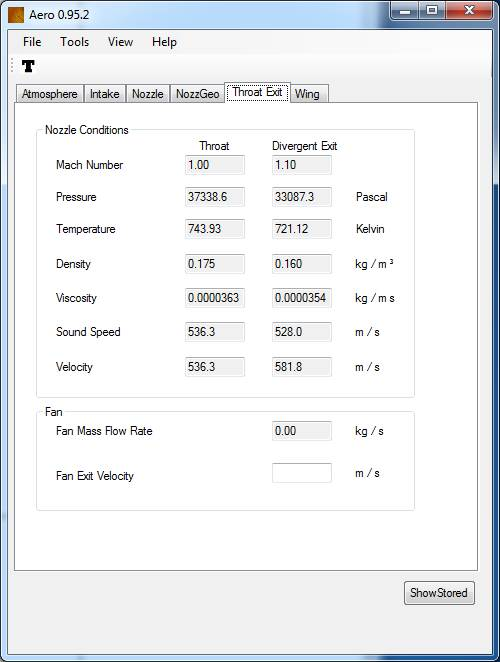The throat and exit nozzle (fully expanded) conditions are given in detail on the fifth tab at the throat and exit.

If the nozzle stagnation conditions are sub critical then the divergent exit fields are left blank.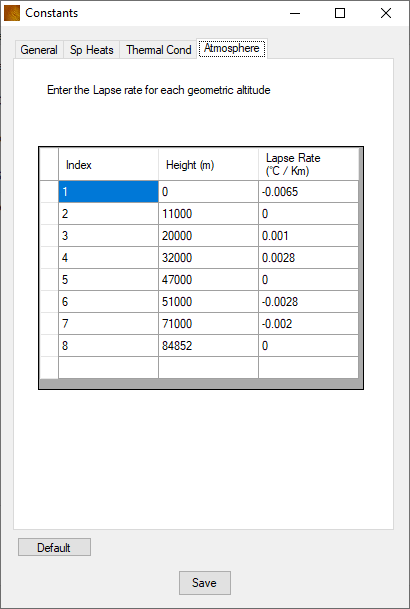Typical constants used within the calculations can be altered.

This is accessed from the top menu bar.

Lapse values can be altered  for each of the altitudes.All the values entered can be saved and re read back into the program using a simple text file. XML can also be exported.Altitude, flight Velocity, Mach, NPR, Net Thrust, Nozzle Stagnation Temperature and Mass Flow rate can all be stored and plotted

These values can also be exported to CSV format.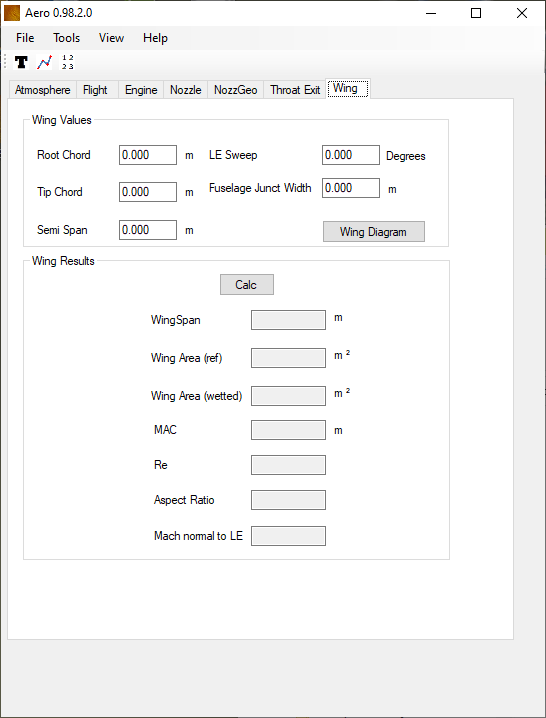A dedicated tab for basic wing  calculations is also included.

Using the data calculated from previous sections the wing Reynolds number and Mach number normal to the leading edge can be returned.